# 隐藏访问策略的属性加密方案研究Attribute-Based Encryption Scheme with Hidden Access Policy

DOI: 10.12677/CSA.2018.83034, PDF, HTML, XML, 下载: 792  浏览: 1,167  科研立项经费支持

Abstract: In the traditional Ciphertext-Policy attributed-based encryption scheme, although the access policy can better implement attribute encryption, it will also bring the problem of data privacy leakage, and the large computation in the Ciphertext decryption stage. This paper proposes an outsourced encryption scheme to hide access policy, which generalizes the values of attributes. In the encryption process, the valid Ciphertext and the invalid Ciphertext are indistinguishable by hiding some subset values. In addition, outsourcing part of the Ciphertext decryption reduces the computing burden of the data receiver and greatly improves the efficiency of decryption. The analysis shows that the new scheme enhances the flexibility of the system while hiding the access policy, and it is proved to be safe under the assumption of DDH, which has certain theoretical and applied value.

1. 引言

2. 预备知识

2.1. 双线性映射

1) 双线性性质。对于任意的 $a,b\in {Z}_{p},\text{\hspace{0.17em}}h\in {G}_{1}$ ，满足 $e\left({g}^{a},{h}^{b}\right)=e{\left(g,h\right)}^{ab}$

2) 非退化性。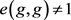3) 可计算性。对于任意的 $g,h\in {G}_{1}$ ,存在有效算法可在多项式时间内计算出 $e\left(g,h\right)$ 的值。

2.2. 访问控制结构

2.3. 安全模型

Init：攻击者向挑战者发送访问控制策略 ${W}_{0}$${W}_{1}$

Setup：挑战者运行初始化过程Setup，将公钥PK发送给攻击者。

Phase 1：攻击者发送属性列表L可以进行多次相关密钥查询，要求L同时满足或者不满足访问控制策略 ${W}_{0}$${W}_{1}$ 。挑战者运行密钥生成算法KeyGen，将密钥 $S{K}_{L}$ 返还给攻击者。

Challenge：攻击者向挑战者提交两个数据 ${M}_{0}$${M}_{1}$ 。如果攻击者的属性列表L在Phase1满足访问策略${W}_{1}$ ，则得到密钥 $S{K}_{L}$ 并要求 ${M}_{0}={M}_{1}$ 。挑战者随机抛币得到 $b\in \left\{0,1\right\}$ ，随机选择一个 ${M}_{b}$ ，用 ${W}_{b}$ 进行加密，将密文信息返回给攻击者。

Phase2：重复Phase1。

Guess：攻击者输出对b的猜测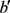3. 具体方案

1) 初始化过程Setup (1k)。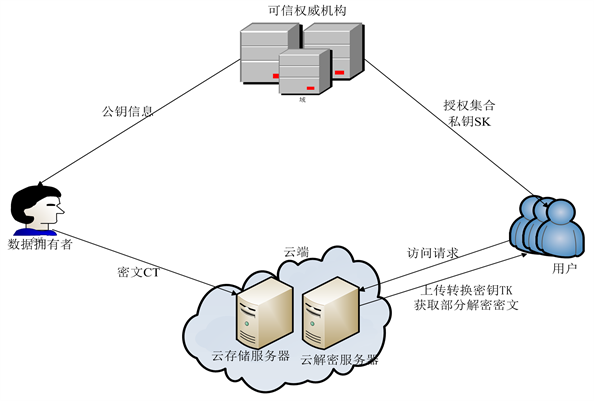Figure 1. System model diagram

2) 加密过程Encrypt ( $PK,M,W$ )。

${C}_{i,t,1}=\left\{\begin{array}{l}{A}_{i,t}^{{s}_{i}},{\nu }_{i,t}\in {W}_{i}\\ random,否则\end{array}$

3) 密钥提取过程KeyGen ( $MK,L$ )。

4) 外包转换计算OutSCC ( $PK,L,TK,CT$ )。

5) 解密过程Decrypt ( $C{T}^{\prime },S{K}_{L}$ )。

4. 安全性分析及证明

4.1. 正确性

1) 验证外包计算。

$\begin{array}{c}\frac{CD}{{\prod }_{i=1}^{n}e\left({C}_{i,1},{D}_{1}\right)}=\frac{{\prod }_{i=1}^{n}e\left({C}_{i,1},{D}_{i,2}\right)}{{\prod }_{i=1}^{n}e\left({g}_{1}^{{s}_{i}},{g}_{2}^{r}\right)}=\frac{e{\left({g}_{1},{g}_{2}\right)}^{rs+{\sum }_{i=1}^{n}{a}_{i,t}\cdot {s}_{i}\cdot {r}_{i}}}{e{\left({g}_{1},{g}_{2}\right)}^{{\sum }_{i=1}^{n}{s}_{i}\cdot r}}\\ =\frac{e{\left({g}_{1},{g}_{2}\right)}^{rs+{\sum }_{i=1}^{n}{a}_{i,t}\cdot {s}_{i}\cdot {r}_{i}}}{e{\left({g}_{1},{g}_{2}\right)}^{rs}}=e{\left({g}_{1},{g}_{2}\right)}^{{\sum }_{i=1}^{n}{a}_{i,t}\cdot {s}_{i}\cdot {r}_{i}}=E\end{array}$

2) 验证解密计算。

$\begin{array}{l}\frac{{C}^{\prime }}{e\left({C}_{0},{D}_{0}\right){\prod }_{i=1}^{n}e\left({C}_{i,t,1},{D}_{i,1}\right)}=\frac{\stackrel{˜}{C}\cdot CD}{e\left({h}^{s},{g}_{2}^{\frac{\alpha +r}{\beta }}\right){\prod }_{i=1}^{n}e\left({A}_{i,t}^{{s}_{i}},{g}_{2}^{{r}_{i}}\right)}\\ =\frac{\stackrel{˜}{C}\cdot {\prod }_{i=1}^{n}e\left({C}_{i,1},{D}_{i,2}\right)}{e\left({g}_{1}^{\beta s},{g}_{2}^{\frac{\alpha +r}{\beta }}\right){\prod }_{i=1}^{n}e\left({g}_{1}^{{a}_{i,t}\cdot s},{g}_{2}^{{r}_{i}}\right)}=\frac{M{Y}^{s}\cdot {\prod }_{i=1}^{n}e\left({C}_{i,1},{D}_{i,2}\right)}{e\left({g}_{1}^{\beta s},{g}_{2}^{\frac{\alpha +r}{\beta }}\right){\prod }_{i=1}^{n}e\left({g}_{1}^{{a}_{i,t}\cdot s},{g}_{2}^{{r}_{i}}\right)}\\ =\frac{M\cdot e{\left({g}_{1},{g}_{2}\right)}^{\alpha s}\cdot e{\left({g}_{1},{g}_{2}\right)}^{rs+{\sum }_{i=1}^{n}{a}_{i,t}\cdot {s}_{i}\cdot {r}_{i}}}{e{\left({g}_{1},{g}_{2}\right)}^{\alpha s+rs}{\prod }_{i=1}^{n}e\left({g}_{1}^{{a}_{i,t}\cdot s},{g}_{2}^{{r}_{i}}\right)}=\frac{M\cdot e{\left({g}_{1},{g}_{2}\right)}^{\alpha s+rs+{\sum }_{i=1}^{n}{a}_{i,t}\cdot {s}_{i}\cdot {r}_{i}}}{e{\left({g}_{1},{g}_{2}\right)}^{\alpha s+rs}e{\left({g}_{1},{g}_{2}\right)}^{{\sum }_{i=1}^{n}{a}_{i,t}\cdot {s}_{i}\cdot {r}_{i}}}\\ =\frac{M\cdot e{\left({g}_{1},{g}_{2}\right)}^{\alpha s+rs+{\sum }_{i=1}^{n}{a}_{i,t}\cdot {s}_{i}\cdot {r}_{i}}}{e{\left({g}_{1},{g}_{2}\right)}^{\alpha s+rs+{\sum }_{i=1}^{n}{a}_{i,t}\cdot {s}_{i}\cdot {r}_{i}}}=M\end{array}$

4.2. 安全性

Proof：假设攻击者A在游戏G0和G中有不可忽略的优势差异e，构造一个能以e的优势打破DDH假设的模拟器B。

Init：模拟器B与攻击者A进行初始化交互。攻击者A选择两个要挑战的密文策略 ${W}_{0}=\left[{W}_{0,1},{W}_{0,2},\cdots ,{W}_{0,n}\right]$ , ${W}_{1}=\left[{W}_{1,1},{W}_{1,2},\cdots ,{W}_{1,n}\right]$ 提交给模拟器B，B随机选取 $u\in \left\{0,1\right\}$

Setup：B任意选取 ${x}^{\prime }\in {Z}_{p}$ ，设置 $\alpha =\frac{ab}{c}+{x}^{\prime }$ ，则 $Y=e{\left({g}_{1},{g}_{2}\right)}^{\frac{ab}{c}+{x}^{\prime }}$ ，对于每个属性 $i\left(1\le i\le n\right)$ ，模拟器B计算 ${A}_{i,t}$ ，计算方法如下：

${A}_{i,t}=\left\{\begin{array}{l}{g}_{1}^{{a}_{i,t}},{\nu }_{i,t}\in {W}_{i,{t}_{i}}\\ {g}_{1}^{b\cdot {a}_{i,t}},{\nu }_{i,t}\notin {W}_{i,{t}_{i}}\end{array}$ ( $1\le t\le n{}_{i}$ )，然后向攻击者A发送公钥PK。

Phase 1：攻击者A在一次密钥查询中提交一个属性列表 $L=\left[{L}_{1},\cdot \cdot \cdot ,{L}_{n}\right]$ ，我们仅考虑L同时不满足 ${W}_{0}$${W}_{1}$ 的情况(如果L同时满足 ${W}_{0}$${W}_{1}$ ，则挑战的信息 ${M}_{0}$${M}_{1}$ 相同，即游戏G和G0就会相同，因此攻击者A在这两个游戏中的优势将没有区别)， $S{K}_{L}$ 中的参数如下： ${D}_{0}={g}_{2}^{\frac{\frac{ab}{c}+{x}^{\prime }+r}{\beta }}$ , ${D}_{1}={g}_{2}^{r}$ ,当 ${L}_{i}\notin {W}_{i}$ 时， ${D}_{i,1}={g}_{2}^{{r}_{i}}$ ，密钥 $S{K}_{L}$ 返回给攻击者A，转换密钥 $TK=\left\{r,{\left\{{D}_{i,1}\right\}}_{1\le i\le n}\right\}$ 发送给外包服务器。

Challenge：攻击者A选择两个挑战信息 ${M}_{0}$${M}_{1}$ 。模拟器B对 ${\left\{{M}_{u}\right\}}_{u\in \left\{0,1\right\}}$ 进行加密，令 $s=c$ ，则密文为： $\stackrel{˜}{C}={M}_{u}{Y}^{s}={M}_{u}e{\left({g}_{1},{g}_{2}\right)}^{\left(\frac{ab}{c}+{x}^{\prime }\right)c}={M}_{u}e{\left({g}_{1},{g}_{2}\right)}^{ab}\cdot e{\left({g}_{1},{g}_{2}\right)}^{{x}^{\prime }c}={M}_{u}e{\left({g}_{1},{g}_{2}\right)}^{c}\cdot e{\left({g}_{1},{g}_{2}\right)}^{{x}^{\prime }c}$${C}_{0}={h}^{s}={h}^{c}$ 。当 $u=0$ 时， $e{\left({g}_{1},{g}_{2}\right)}^{c}=e{\left({g}_{1},{g}_{2}\right)}^{ab}$ ；当 $u=1$ 时， $e{\left({g}_{1},{g}_{2}\right)}^{c}$ 为随机值，因此， $\stackrel{˜}{C}$${G}_{T}$ 群中的随机值。模拟器B选取随机值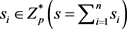，计算 ${\left\{{C}_{i,t,1}\right\}}_{1\le t\le {n}_{i}}={A}_{i,t}^{{s}_{i}}={g}_{1}^{b\cdot {a}_{i,t}\cdot {s}_{i}}$${C}_{i,1}={g}_{1}^{{s}_{i}}$ ，把密文 $CT=\left\{\stackrel{˜}{C},{C}_{0},{\left\{{C}_{i,1},{\left\{{C}_{i,t,1}\right\}}_{1\le t\le {n}_{i}}\right\}}_{1\le i\le n}\right\}$ 发送给外包服务器，外包服务器通过转换密钥TK将密文CT进行转换，首先计算 $CD={\prod }_{i=1}^{n}e\left({C}_{i,1},{D}_{i,2}\right)=e{\left({g}_{1},{g}_{2}\right)}^{rs+{\sum }_{i=1}^{n}b\cdot {a}_{i,t}\cdot {s}_{i}\cdot {r}_{i}}=e{\left({g}_{1},{g}_{2}\right)}^{rc+{\sum }_{i=1}^{n}b\cdot {a}_{i,t}\cdot {s}_{i}\cdot {r}_{i}}$$1\le t\le {n}_{i}$，然后将转换后的密文 ${C}^{\prime }=\stackrel{˜}{C}\cdot CD$ 发送给攻击者A。

Phase 2：重复Phase 1的过程，继续进行私钥问询。

Guess：攻击者A输出对u的猜测 ${u}^{\prime }$ 。如果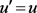,挑战者输出1；否则，输出0。通过我们之前的假设，攻击者A在游戏G中猜测正确u的可能性相比在游戏G0中正确猜出u有e的优势。当 $e{\left({g}_{1},{g}_{2}\right)}^{c}=e{\left({g}_{1},{g}_{2}\right)}^{ab}$ 时，攻击者A猜测游戏为G；当 $e{\left({g}_{1},{g}_{2}\right)}^{c}$ 是随机值时，攻击者A猜测游戏为G0。因此，模拟器B在DDH游戏中有e的优势，即最后攻破了DDH问题。

5. 性能分析

1) 隐藏访问控制策略。本方案对每个属性的取值进行泛化，增加其可能取值，通过隐藏每个属性包含部分可能取值的子集，使用通配符表示那些与访问策略不相关的属性，如此便达到了隐藏部分策略属性的目的。保证了包括合法接收方在内的所有接收方都不能猜测出加密时采用的访问控制策略，而接收方需要从可信授权方获取自己的属性私钥，若与此属性私钥相关联的属性列表不满足发送方指定的访问策略时，则此接收方就不是合法的，不能完成解密。

2) 支持访问结构中属性的动态添加。对比文献  和  中要求密文中的访问策略在初始化之前就指定好属性个数和每个属性的取值且不能随意更改访问策略中相关属性的信息；文献  中，首先执行初始化算法，然后在密文中的访问策略中添加新属性。由于公共参数没有变，运行初始化算法Setup之后，接收方已经可以获得用户私钥，这时再在访问策略中添加属性，对于某些用户来说仍然具备解密能力。例如，访问策略 $W=\left[{W}_{1},{W}_{2},{W}_{3}\right]$ ，用户属性列表为 $L=\left[{L}_{1},{L}_{2},{L}_{3}\right]=\left[1,1,0\right]$ ，与用户属性列表相关联的密钥是 $S{K}_{L}$ ，若改变访问策略使其变为 $W=\left[{W}_{1},{W}_{2},{W}_{3},{W}_{4}\right]$ ，这时要求合法的解密者拥有的密钥 $S{K}_{L}$ 必须具有 ${W}_{4}$ 相关属性成分，但是对于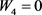来说，由于L仍然满足W，因此某些用户仍然具备解密能力。

3) 提高系统效率。整个系统运行过程中，影响效率的主要因素体现在存储开销和系统计算时间上。为了方便下文的分析描述，令 $|g|,|{g}_{T}|$$|p|$ 分别代表  中元素的大小，n表示系统中的属性个数，N代表n个属性的所有可能取值个数之和， ${\Gamma }_{e}$ 表示执行一次双线性运算所需要的时间， ${\Gamma }_{\Delta }$ 表示执行一次指数运算花费的时间， $|{A}_{c}|$ 表示访问控制策略中所包含的属性个数。Table 2. Computation of time contrast

6. 结语

  Shamir, A. (1984) Identity-Based Cryptosystems and Signature Schemes. Proceedings of CRYPTO’84 on Advances in Cryptology, 21, 47-53.  Sahai, A. and Waters, B. (2005) Fuzzy Identity Based Encryption. Advances in Cryptology-EUROCRYPT 2005, 3494, 457-473. https://doi.org/10.1007/11426639_27  冯登国, 陈成. 属性密码学研究[J]. 密码学报, 2014, 1(1): 1-12.  苏金树, 曹丹, 王小峰. 属性基加密机制[J]. 软件学报, 2011, 22(6): 1299-1315.  Goyal, V., Pandey, O., Sahai, A. and Waters, B. (2006) Attribute-Based Encryption for Fine-Grained Access Control of Encrypted Data. Proceedings of the ACM Conference on Computer and Communications Security, October 30-November 3 2006, Alexandria, Virginia, 89-98. https://doi.org/10.1145/1180405.1180418  Cheung, L. and Newport, C. (2007) Provably Secure Ciphertext Policy ABE. CCS’07: Proceedings of the ACM Conference on Computer and Communications Security, Alexandria, Virginia, 456-465. https://doi.org/10.1145/1315245.1315302  Bethencourt, J., Sahai, A. and Waters, B. (2007) Ciphertext-Policy Attrib-ute-Based Encryption. Proceedings of the IEEE Symposium on Security and Privacy, 20-23 May 2007, Washington, DC, 321-334. https://doi.org/10.1109/SP.2007.11  Emura, K., Miyaji, A. and Nomura, A. (2009) A Ciphertext-Policy Attribute Based En-cryption Scheme with Constant Ciphertext Length. ISPEC 2009: Information Security Practice and Experience, 5451, 13-23. https://doi.org/10.1007/978-3-642-00843-6_2  Nishide, T., Yoneyama, K. and Ohta, K. (2008) Attribute-Based Eneryption with Partially Hidden Encryption-Specified Access Structure. Proceedings of the Applied Cryptograph & Network Security, 5037, 111-129.  Kapadi, A., Tsang, P. and Smith, W. (2007) Attribute-Based Publishing with Hidden Credentials and Hidden Policies. Network & Distributed System Security Symposium, 179-192.  Shi, E., Bethncourt, J., Chan, S. and Perrig, A. (2007) Mul-ti-Dimensional Range Query over Encrypted Data. Proceedings of IEEE Symposium on Security and Privacy, 20-23 May 2007, Berke-ley, CA, 350-364. https://doi.org/10.1109/SP.2007.29  Bonehd, D. and Waters, B. (2007) Conjunctive, Subset, and Range Queries on Encrypt-ed Data. In: Vadhan, S.P., Ed., TCC 2007, Vol. 4392, 535-554.  Lai, J., Deng, R.H. and Li, Y. (011) B-Ktlly Secure Ciper-text-Policy Hiding CP-ABE. ISPEC 2011, 6672, 24-39．  Katz, J., Sahai, A. and Waters, B. (2008) Predicate Encryption Sup-porting Disjunctions, Polynomial Equations, and Inner Products. Advances in Cryptology-EUROCRYPT 2008, 4965, 146-162. https://doi.org/10.1007/978-3-540-78967-3_9